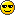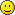RETROBAT Board
Would you like to react to this message? Create an account in a few clicks or log in to continue.

# [OBSOLETE] My solution (Autohotkey) : Close external emulators with xbox one controller

2 posters##[OBSOLETE] My solution (Autohotkey) : Close external emulators with xbox one controller

In retroarch its generally start+select to exit a core, but using external emulators, pcsx2.exe for example you can hotkey the ESC to exit a controller, but requires a keyboard.

Heres my solution exit an emulator using the controller only. (pcsx2.exe) Can add as many as you like!...Install autohotkey and put these 2 files in same directory.

Usage: we hold down LB+RB for around 10/15 seconds to close the pcsx2.exe, sending us back into emulationstation. The script will loop for us until we close it.

Add multiple lines for different emulators e.g.

Process, Close, pcsx2.exe
Process, Close, emulator1.exe
Process, Close, emulator2.exe
Process, Close, emulator3.exe

Save close_emulator.ahk

Code:
`#include XInput.ahkSetTitleMatchMode,2Min(x,x1="",x2="",x3="",x4="",x5="",x6="",x7="",x8="",x9="") {   Loop      IfEqual x%A_Index%,, Return x      Else x := x < x%A_Index% ? x : x%A_Index%}Max(x,x1="",x2="",x3="",x4="",x5="",x6="",x7="",x8="",x9="") {   Loop      IfEqual x%A_Index%,, Return x      Else x := x > x%A_Index% ? x : x%A_Index%}MySendInput(i) { If WinActive("craymachine"){ sendinput % Chr(i) }}; Example: Control the vibration motors using the analog triggers of each controller.XInput_Init()oVar := 0count := 0Loop { Loop, 15 { dt := 10000 if State := XInput_GetState(A_Index-1) { LT := State.bLeftTrigger RT := State.bRightTrigger buttons := State.wButtons if(LT > 0 || RT > 0){ ;XInput_SetState(A_Index-1, LT*257, RT*257)  } if(LT > 1 && RT > 1){ Buttonspressed += 1 ; adds 1 to Buttonspressed ;XInput_SetState(A_Index-1, LT*257, RT*257) } else if (abs(State.sThumbLX) > dt || abs(State.sThumbLY) > dt) {  } else if (abs(State.sThumbRX) > dt || abs(State.sThumbRY) > dt) {  } else { XInput_SetState(A_Index-1, 0, 0) }  if(buttons & XINPUT_GAMEPAD_A){ if(count < 20){ MySendInput(oVar) } else if(count == 30){ Loop 30 { MySendInput(oVar) Sleep, 10 } oVar = 0 } else if(count == 48 && buttons & XINPUT_GAMEPAD_DPAD_UP){ Random, oVar, 97, 122 MySendInput(oVar) } else if(count == 50 && buttons & XINPUT_GAMEPAD_DPAD_UP){ count = 29 } else if(count > 30){ ; Do Nothing } count+=1 } else if(buttons > 0) { ; arr := ["a","b","c","d","e","f","g","h","i","j","k","l","m","n","o","p","q","r","s","t","u","v","w","x","y","z"] Random, oVar, 97, 122 ; \$q:: ;Random, oVar, 1, 4  ; sendinput % arr[oVar] MySendInput(oVar) ; return  ; lastVar = oVar ; lastButtons = buttons count = 0 } } } Sleep, 300 if(Buttonspressed > 20){ Process, Close, pcsx2.exe Buttonspressed = 0 ; reset }}`

xinput.ahk

Code:
`/*  XInput by Lexikos *  This version of the script uses objects, so requires AutoHotkey_L. *//*    Function: XInput_Init        Initializes XInput.ahk with the given XInput DLL.        Parameters:        dll     -   The path or name of the XInput DLL to load.*/XInput_Init(dll="xinput1_3"){    global    if _XInput_hm        return        ;======== CONSTANTS DEFINED IN XINPUT.H ========        ; NOTE: These are based on my outdated copy of the DirectX SDK.    ;       Newer versions of XInput may require additional constants.        ; Device types available in XINPUT_CAPABILITIES    XINPUT_DEVTYPE_GAMEPAD          := 0x01    ; Device subtypes available in XINPUT_CAPABILITIES    XINPUT_DEVSUBTYPE_GAMEPAD       := 0x01    ; Flags for XINPUT_CAPABILITIES    XINPUT_CAPS_VOICE_SUPPORTED     := 0x0004    ; Constants for gamepad buttons    XINPUT_GAMEPAD_DPAD_UP          := 0x0001    XINPUT_GAMEPAD_DPAD_DOWN        := 0x0002    XINPUT_GAMEPAD_DPAD_LEFT        := 0x0004    XINPUT_GAMEPAD_DPAD_RIGHT       := 0x0008    XINPUT_GAMEPAD_START            := 0x0010    XINPUT_GAMEPAD_BACK             := 0x0020    XINPUT_GAMEPAD_LEFT_THUMB       := 0x0040    XINPUT_GAMEPAD_RIGHT_THUMB      := 0x0080    XINPUT_GAMEPAD_LEFT_SHOULDER    := 0x0100    XINPUT_GAMEPAD_RIGHT_SHOULDER   := 0x0200    XINPUT_GAMEPAD_A                := 0x1000    XINPUT_GAMEPAD_B                := 0x2000    XINPUT_GAMEPAD_X                := 0x4000    XINPUT_GAMEPAD_Y                := 0x8000    ; Gamepad thresholds    XINPUT_GAMEPAD_LEFT_THUMB_DEADZONE  := 7849    XINPUT_GAMEPAD_RIGHT_THUMB_DEADZONE := 8689    XINPUT_GAMEPAD_TRIGGER_THRESHOLD    := 30    ; Flags to pass to XInputGetCapabilities    XINPUT_FLAG_GAMEPAD             := 0x00000001        ;=============== END CONSTANTS =================        _XInput_hm := DllCall("LoadLibrary" ,"str",dll)        if !_XInput_hm    {        MsgBox, Failed to initialize XInput: %dll%.dll not found.        return    }        _XInput_GetState        := DllCall("GetProcAddress" ,"ptr",_XInput_hm ,"astr","XInputGetState")    _XInput_SetState        := DllCall("GetProcAddress" ,"ptr",_XInput_hm ,"astr","XInputSetState")    _XInput_GetCapabilities := DllCall("GetProcAddress" ,"ptr",_XInput_hm ,"astr","XInputGetCapabilities")        if !(_XInput_GetState && _XInput_SetState && _XInput_GetCapabilities)    {        XInput_Term()        MsgBox, Failed to initialize XInput: function not found.        return    }}/*    Function: XInput_GetState        Retrieves the current state of the specified controller.    Parameters:        UserIndex   -   [in] Index of the user's controller. Can be a value from 0 to 3.        State       -   [out] Receives the current state of the controller.        Returns:        If the function succeeds, the return value is ERROR_SUCCESS (zero).        If the controller is not connected, the return value is ERROR_DEVICE_NOT_CONNECTED (1167).        If the function fails, the return value is an error code defined in Winerror.h.            http://msdn.microsoft.com/en-us/library/ms681381.aspx    Remarks:        XInput.dll returns controller state as a binary structure:            http://msdn.microsoft.com/en-us/library/microsoft.directx_sdk.reference.xinput_state            http://msdn.microsoft.com/en-us/library/microsoft.directx_sdk.reference.xinput_gamepad        XInput.ahk converts this structure to an AutoHotkey_L object.*/XInput_GetState(UserIndex){    global _XInput_GetState        VarSetCapacity(xiState,16)    if ErrorLevel := DllCall(_XInput_GetState ,"uint",UserIndex ,"uint",&xiState)        return 0        return {    (Join,        dwPacketNumber: NumGet(xiState,  0, "UInt")        wButtons:       NumGet(xiState,  4, "UShort")        bLeftTrigger:   NumGet(xiState,  6, "UChar")        bRightTrigger:  NumGet(xiState,  7, "UChar")        sThumbLX:       NumGet(xiState,  8, "Short")        sThumbLY:       NumGet(xiState, 10, "Short")        sThumbRX:       NumGet(xiState, 12, "Short")        sThumbRY:       NumGet(xiState, 14, "Short")    )}}/*    Function: XInput_SetState        Sends data to a connected controller. This function is used to activate the vibration    function of a controller.        Parameters:        UserIndex       -   [in] Index of the user's controller. Can be a value from 0 to 3.        LeftMotorSpeed  -   [in] Speed of the left motor, between 0 and 65535.        RightMotorSpeed -   [in] Speed of the right motor, between 0 and 65535.        Returns:        If the function succeeds, the return value is 0 (ERROR_SUCCESS).        If the controller is not connected, the return value is 1167 (ERROR_DEVICE_NOT_CONNECTED).        If the function fails, the return value is an error code defined in Winerror.h.            http://msdn.microsoft.com/en-us/library/ms681381.aspx        Remarks:        The left motor is the low-frequency rumble motor. The right motor is the        high-frequency rumble motor. The two motors are not the same, and they create        different vibration effects.*/XInput_SetState(UserIndex, LeftMotorSpeed, RightMotorSpeed){    global _XInput_SetState    return DllCall(_XInput_SetState ,"uint",UserIndex ,"uint*",LeftMotorSpeed|RightMotorSpeed<<16)}/*    Function: XInput_GetCapabilities        Retrieves the capabilities and features of a connected controller.        Parameters:        UserIndex   -   [in] Index of the user's controller. Can be a value in the range 03.        Flags       -   [in] Input flags that identify the controller type.                                0   - All controllers.                                1   - XINPUT_FLAG_GAMEPAD: Xbox 360 Controllers only.        Caps        -   [out] Receives the controller capabilities.        Returns:        If the function succeeds, the return value is 0 (ERROR_SUCCESS).        If the controller is not connected, the return value is 1167 (ERROR_DEVICE_NOT_CONNECTED).        If the function fails, the return value is an error code defined in Winerror.h.            http://msdn.microsoft.com/en-us/library/ms681381.aspx        Remarks:        XInput.dll returns capabilities via a binary structure:            http://msdn.microsoft.com/en-us/library/microsoft.directx_sdk.reference.xinput_capabilities        XInput.ahk converts this structure to an AutoHotkey_L object.*/XInput_GetCapabilities(UserIndex, Flags){    global _XInput_GetCapabilities        VarSetCapacity(xiCaps,20)        if ErrorLevel := DllCall(_XInput_GetCapabilities ,"uint",UserIndex ,"uint",Flags ,"ptr",&xiCaps)        return 0        return,    (Join        {            Type:                   NumGet(xiCaps,  0, "UChar"),            SubType:                NumGet(xiCaps,  1, "UChar"),            Flags:                  NumGet(xiCaps,  2, "UShort"),            Gamepad:            {                wButtons:           NumGet(xiCaps,  4, "UShort"),                bLeftTrigger:       NumGet(xiCaps,  6, "UChar"),                bRightTrigger:      NumGet(xiCaps,  7, "UChar"),                sThumbLX:           NumGet(xiCaps,  8, "Short"),                sThumbLY:           NumGet(xiCaps, 10, "Short"),                sThumbRX:           NumGet(xiCaps, 12, "Short"),                sThumbRY:           NumGet(xiCaps, 14, "Short")            },            Vibration:            {                wLeftMotorSpeed:    NumGet(xiCaps, 16, "UShort"),                wRightMotorSpeed:   NumGet(xiCaps, 18, "UShort")            }        }    )}/*    Function: XInput_Term    Unloads the previously loaded XInput DLL.*/XInput_Term() {    global    if _XInput_hm        DllCall("FreeLibrary","uint",_XInput_hm), _XInput_hm :=_XInput_GetState :=_XInput_SetState :=_XInput_GetCapabilities :=0}; TODO: XInputEnable, 'GetBatteryInformation and 'GetKeystroke.`zack0

Messages : 4
Date d'inscription : 2020-05-18

THIAGO GONÇALVES likes this post

##Re: [OBSOLETE] My solution (Autohotkey) : Close external emulators with xbox one controller

Hello,
thank you, but in the v3.0 final version, it will not be necessary.Lorenzolamas